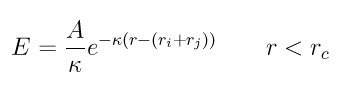# [lammps-users] DLVO parameter setting

Dear lammps users,

I am using the DLVO yukawa/colloid pair style and having a problem with the parameter settings.

According to the manual, the pairwise interaction is calculated according to this equation``````where A = 2*PI*eps*eps0*kappa * R * psi^2;        and eps*eps0 * kappa are constants.
``````

It is known that for particle i, the radius is 6 nm and psi is +22mV; for Particle j, the radius is 20 nm and the psi is -10mV;

Based on these, how can I calculate the parameter A between i and j? Especially, the psi is in the different negative -10mV and positive +22mV.

Best regards

Ting